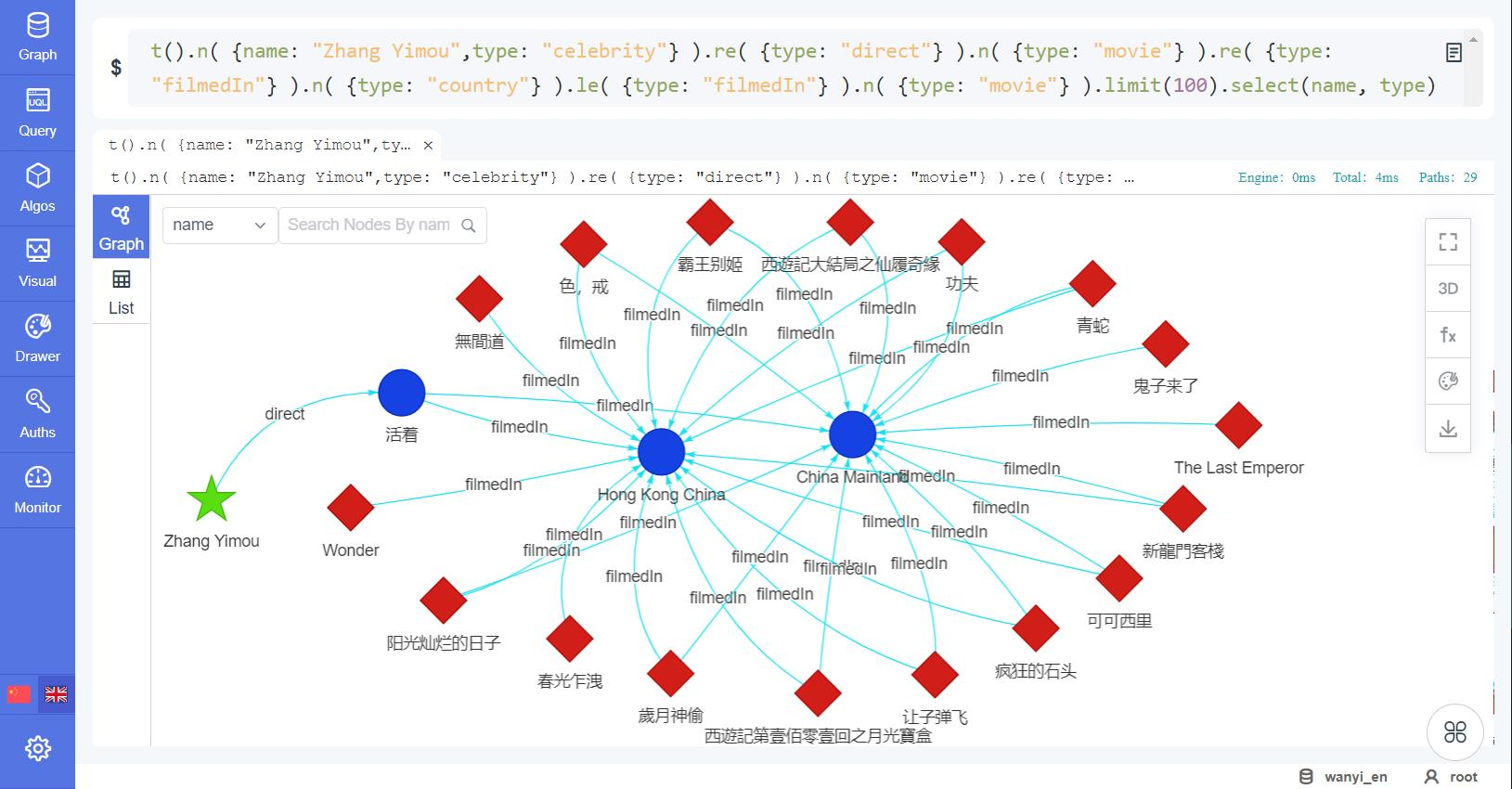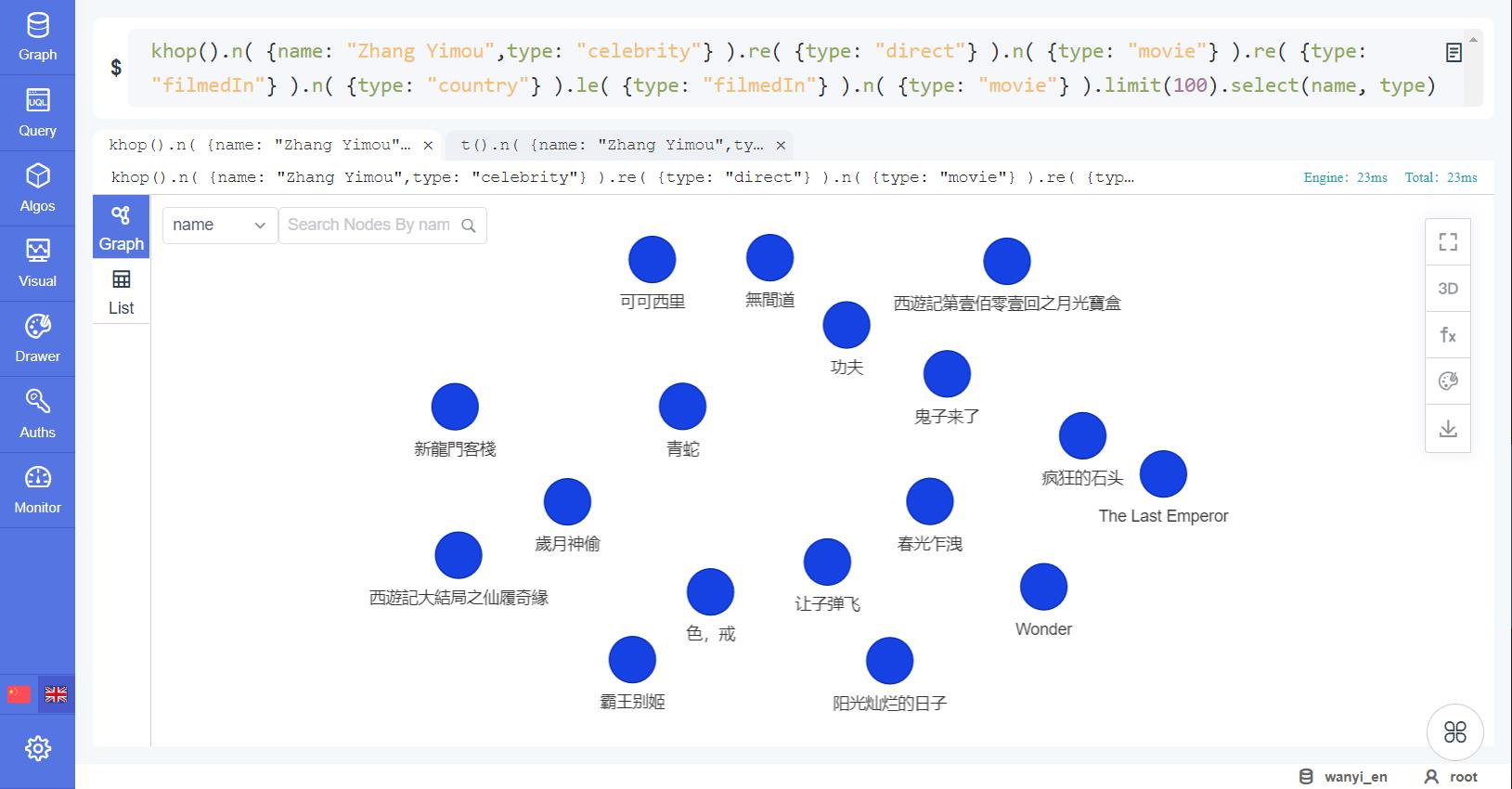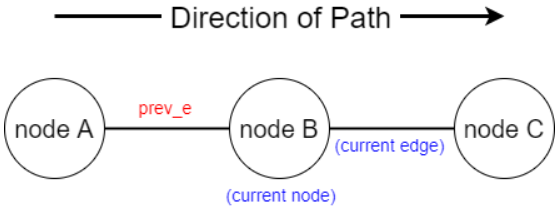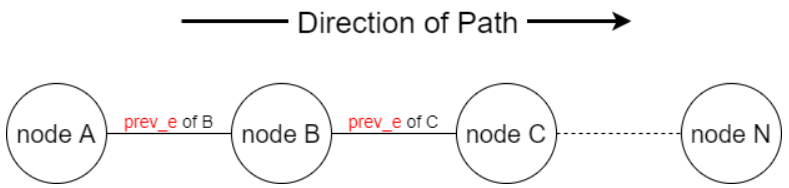# Change Nickname

Current Nickname:
Search
v2.x
v2.x

# Template Based Query

Template-based query or shortened as template query is best described as an upgraded version of those subgraph extraction methods discussed in the previous chapter. It queries against the graphset with much more flexible and precise filtering abilities to find paths and neighbors.

In one word, template queries that use a set of advanced parameters, called 'template' and repeatable in an uQL, are able to segment the path into sub-paths and apply filters separately. For instance, a path that can only be filtered against 'all 4 edges are left directing' in an A-B query, now can be filtered against 'the first 2 edges are left directing and the last 2 are right directing' in a template query.

More advantages of template query over previous queries are listed:

• specifying starting/ending nodes using Ultipa filter other than Ultipa ID;
• introducing alias to enable inter-step filtering that is more diverse than just 'ascending/descending';
• supporting various types of returns, like path, meta-data, properties, aggregations, etc.;
• ...

By leveraging template queries, Ultipa users can accommodate their business needs with celerity and great flexibility.

Examples in this chapter can be validated by running against a test graphset with meta-data information provided: node_info,edge_info

## uQL Components

The uQL components of template query:

Type Components
Command `t(<>)` or `khop()`
Parameter `n(<>)`, `nf(<>)`, `e(<>)`, `le(<>)`, `re(<>)`, `[]`,
`no_circle(<>)`,
`order_by(<>)`, `group_by(<>)`,
`limit(<>)`
Return (no alias:) `select(<>)` or `select_node_properties(<>)` or `select_edge_properties(<>)`,
(with alias:) `return(<>)` ,
total number of results (for `khop()` only)

Where:

• `t(<>)` is to find paths using template query;
• `khop()` is to find neighbors using template query.

Values of parameter and return:

Name Data Type Specification Description
n obj Ultipa filter (Repeatable) The starting/ending node of sub-path
nf obj Ultipa filter (Repeatable) The in-between nodes other than the starting/ending nodes of sub-path
e obj Ultipa filter (Repeatable) The edges of sub-path
le obj Ultipa filter (Repeatable) The edges of sub-path all of which are left directing
re obj Ultipa filter (Repeatable) The edges of sub-path all of which are right directing
[ ] int; range sum of all depths<30 The number of edges in the sub-path;
`[N]`: N edges
`[:N]`: 1~N edges (for `t()` only)
`[N:M]`: N~M edges (for `t()` only)
`[*:N]`: the number of edges of the shortest sub-path within N steps (for `t()` only)
no_circle / / (Optional) To dismiss the paths with circles
order_by obj (see aggregation section) (Optional) To order the results by specific rules
group_by string (see aggregation section) (Optional) To group the results by specific value
limit int >0; -1 (Optional) The maximum number of results to return; -1: return all the results
select []string comma (,) separated; * (1/2, optional) A list of properties to return; *: return all the properties; does not coexist with `select_node_properties()` or `select_edge_properties()`
select_node_properties []string comma (,) separated; * (1/4, optional) A list of node properties to return; *: return all the node properties; does not coexist with `select()`
select_edge_properties []string comma (,) separated; * (1/4, optional) A list of edge properties to return; *: return all the edge properties; does not coexist with `select()`
return / comma (,) separated (see return section) (Optional) A list of path, meta-data, properties or aggregations to return

Where:

• when `limit()` is not included in the uQL, it's considered as `limit(3)`;
• _id, _from_id and _to_id are the default properties to be returned and need not to be declared in `select()`, `select_node_properties()` and `select_edge_properties()`.

## Syntax w/o Alias

### `t()...select()`

Syntax of a typical path-finding template query:

``````t()
.n(<>).e(<>).nf(<>)[<int | range>].n(<>)
.e(<>).nf(<>)[<int | range>].n(<>)
.e(<>).nf(<>)[<int | range>].n(<>)
...
.limit(<int>).select(<string>, <string>, ...)
``````

The uQL shown above is wrapped into sub-paths in separated lines for purpose of easy reading, where:

• `<filter>` is written as `<>` for short;
• only the 1st sub-path has `n()` for both starting node and ending node, the 2nd and later have only `n()` for ending node;
• any `e()` can be replaced by `le()` or `re()` if edge direction is to be restricted in the respective sub-path;
• any `nf()` can be omitted if no filtering rules against the in-between nodes are to be applied in the respective sub-path;
• `nf().` or an `nf()` with no `.[]` following is meaningless and will be neglected by the system.

### `khop()...select()`

Syntax of neighbor-finding template query can be derived from that of path-finding template query, by replacing `t()` with `khop()` and restricting sub-path depth to fixded interger:

``````khop()
.n(<>).e(<>).nf(<>)[<int>].n(<>)
.e(<>).nf(<>)[<int>].n(<>)
.e(<>).nf(<>)[<int>].n(<>)
...
.limit(<int>).select(<string>, <string>, ...)
``````

### Examples

Example 1: Starting from nodes (forum users) where industry = 'Education', search for the shortest path to nodes where industry = 'IT', the maximal depth is 5

``````t()
.n( {industry:"Education"} )
.e()[*:5]
.n( {industry:"IT"} )
.limit(10).select(*)
``````

Example 2: Based on the solution of Example 2, all the in-between nodes need to be female users, and all the edges are the 'agree' type.

``````t()
.n( {industry: "Education"} )
.e( {type:"agree"} ).nf( {gender:"female"} )[*:5]
.n( {industry: "IT"} )
.limit(10).select(*)
``````

Example 3: Starting from the node of celebrity Zhang Yimou search for 3-step paths, firstly to the movies directed by him, then to the countries where these movies were filmed, finally to the movies that were shot in these countries, return names of movie and types of edge

``````t()
.n( {name: "Zhang Yimou",type: "celebrity"} )
.re( {type: "direct"} ).n( {type: "movie"} )
.re( {type: "filmedIn"} ).n( {type: "country"} )
.le( {type: "filmedIn"} ).n( {type: "movie"} )
.limit(100).select(name, type)
``````

Running Example 3 within Ultipa-Manager will get this:Figure: t() template Query of movies

Many ending nodes in the above screenshot are reachable via multiple paths. To abstract these ending nodes with NO repetition, either use neighbor-finding template query, or use aggregation which will be introduced later in this chapter.

Example 4: Same as Example 3, but using `khop()` command, which is to return movies that were filmed in the same locations as those directed by Zhang Yimou

``````khop()
.n( {name: "Zhang Yimou",type: "celebrity"} )
.re( {type: "direct"} ).n( {type: "movie"} )
.re( {type: "filmedIn"} ).n( {type: "country"} )
.le( {type: "filmedIn"} ).n( {type: "movie"} )
.limit(100).select(name)
``````

Running Example 4 within Ultipa-Manager will get this:Figure: khop() template Query of movies

## Syntax with Alias

### Alias

Alias is a convenient feature that only exists with path-finding template queries, via which the meta-data and paths can be referred to AFTER being defined, for operational purposes of filtering, aggregation, or simply just declaring information to be returned.

Two types of alias are supported:

• the system reserved alias: `prev_n` and `prev_e`, aforementioned at the end of chapter Background;
• the user-defined alias.

The naming convention for user-defined alias:

• Only includes underscore (_), numbers [0-9] or letters [a-zA-Z]
• Length between [1, 64] ASCII characters
• Should NOT use 'prev_n' or 'prev_e'
• Should NOT repeat within one uQL

### `t()...return()`

Syntax of path-finding template query with user-defined alias, taking example of a 1-step sub-path and a multi-step sub-path:

``````t(<alias>)
.n(<alias><>).e(<alias><>).n(<alias><>)
.e(<>).nf(<>)[<int | range>].n(<alias><>)
...
.limit(<int>).return(<info>, ..., <aggregation> as <aliasAgg>, ...)
``````

Where:

• `<filter>` is written as `<>` for short;
• any `e()` can be replaced by `le()` or `re()` if edge direction is to be restricted in the respective sub-path;
• `t()` (so is path) and `n()` can always have alias defined;
• `nf()` can never be defined with alias;
• `e()`, `le()` or `re()` in the 1-step sub-path can have alias;
• aggregations with results of a single value can have secondary alias defined via 'as' when they are being declared in `return()`, for ordering purposes.

Note: System reserved aliases are used for filtering purpose only, they appear only in `<filter>`, and are not shown in detail in the above uQL; user-defined alias has a wider range of applications, and can be found in `<filter>`, `return()`, `group_by()` and `order_by()`.

### Examples

Example: Find 1-step paths from nodes of forum users born in the 1990s to movies that they added to the wishlist, return paths, names of these movies, and return the number of movies as 'movieCount'

``````t(p)
.n( {type:"account", year: {\$bt:[1990,1999]} } )
.e( {type:"wishlist"} ).n(n {type:"movie"} )
.limit(5).return(p, n.name, {\$count_distinct: n} as movieCount)
``````

## Return Info

Declaring information in `return()` is the most general usage of user-defined alias.

### `return(<path>)`

Given the path alias defined in `t()`, path and properties can be returned.

To return path:

``````return(<alias>)
``````

To return properties respectively:

``````return(<alias> {<node_properties>} {<edge_properties>} )
``````

To return properties with common property names:

``````return(<alias> {<properties>} )
``````

Where:

Value Data Type Specification Description
`<node_properties>` []string comma (,) separated node properties; * A list of node properties to return; *: return all the node properties
`<edge_properties>` []string comma (,) separated edge properties; * A list of edge properties to return; *: return all the edge properties
`<properties>` []string comma (,) separated properties; * A list of common properties to return; *: return all the properties

### `return(<node> | <edge>)`

Given the node alias defined in `n()` or `nf()`, or edge alias defined in `e()` or `le()` or `re()`, the meta-data and properties can be returned.

To return meta-data:

``````return(<alias>)
``````

To return properties:

``````return(<alias>.<property>, <alias>.<property>, ...)
``````

## Inter-Step Filter

Inter-step filter, as the name suggests, is to filter current meta-data against the property value of other meta-data in the path. It is a type of filter that only exists in templates.

Inter-step filter works in a backward matching pattern, meaning that the current meta-data will be filtered against the property value of its previous meta-data, which is referenced by either system reserved alias or user-defined alias.

### `prev_e`

`prev_e` is a system reserved alias that refers to the 'previous edge' of the current meta-data in a path. Compared with user-defined alias, it has the ability to dynamically point at the edge right before the current meta-data in the path.Figure: Demonstration on prev_e

Example 1: Given user reviews of movies and user responses, search for 2-step paths from movies to users, in which the 1st user reviews a movie, and the 2nd user responses to the review of the 1st user.

``````t(p)
.n({type: "movie"})
.le({type: "review"}).n()
.le({type: "response", target_post: prev_e._id}).n()
.limit(100).return(p {*})
``````

`prev_e` also works in recursive fashion, which feature greatly helps in the query of paths with sequential internal relations.Figure: Demonstration on prev_e in Recursion

Example 2: Same as Example 1, but search until the 5th~7th users that give responses.

``````t(p)
.n({type: "movie"})
.le({type: "review"}).n()
.le({type: "response", target_post: prev_e._id})[5:7].n()
.limit(100).return(p {*})
``````

Being able to search in a recursive fashion is one salient advantage of graph computing query language, and Ultipa aims to offer the most advanced recursive graph query language features to the customers. Stay tuned for our continuous advancement.

### `prev_n`

`prev_n` is another system reserved alias and it refers to the 'previous node' of the current meta-data in a path, and works in a way similar to `prev_e`.

Example: Revise the previous example, eliminate the situations in which the same user responses to himself (or 'no circle').

``````t(p)
.n({type: "movie"})
.le({type: "review"}).n()
.le({type: "response", target_post: prev_e._id})
.nf({_id: {\$neq: prev_n._id}})[5:7]
.n({_id: {\$neq: prev_n._id}})
.limit(100).return(p {*})
``````

### `alias` (User-Defined)

The backward matching pattern requires the alias to be already defined before being referred to.

Example: Given user reviews of movies and user opinions, search for 3-step circular paths from movies to themselves, in which the 1st user reviews a movie, then the 2nd user does not agree with the 1st user and later reviews on the same movie by himself.

``````t(p)
.n(a {type: "movie"})
.le({type: "review"}).n()
.le({type: "disagree", target_post: prev_e._id}).n()
.re({type: "review", timestamp: {\$gt: prev_e.timestamp}})
.n({_id: a._id})
.limit(10).return(p {*})
``````

## Aggregation

Ultipa aggregations are mathematical functions comprising operator and operand, which are used to aggregate the query result.

In a traditional database, the aggregation function is used to 'group' values of multiple rows to form a single summary value. In Ultipa Graph, aggregation works against operands (so are path, meta-data and properties) referenced via user-defined aliases.

`Note: For any property to be processed in aggregation operation, LTE it first.`

Syntax of aggregation is:

``````{ <operator> : <operand> }
``````

Ultipa aggregation introduces the following operators:

Operator Encapsulator Meaning
\$sum `return()` sums the operand values (numeric property) in each group of query results
\$avg `return()` averages the operand values (numeric property) in each group of query results
\$min `return()` picks the minimal operand value (numeric property) in each group of query results
\$max `return()` picks the maximal operand value (numeric property) in each group of query results
\$collect `return()` picks the distinct operand values in each group of query results
\$count_distinct `return()` counts the distinct operand values in each group of query results
/ `group_by()` groups the query results by operand
/ `order_by()` orders the query results by operand, or orders the groups by their aggregation results

`Note: The last two aggregation operations do not involve operators; they have their own syntax different than above.`

### `\$sum`

To sum the values of numeric property:

``````{ \$sum : <alias>.<property> }
``````

Example: Calculate the sum of number of words of reviews of movie La vita è bella‎ ( id = 1006 )

``````t()
.n(1006).le(b {type: "review"} ).n( {type: "account"} )
.return( {\$sum: b.value} )
``````

### `\$avg`

To average the values of numeric property:

``````{ \$avg : <alias>.<property> }
``````

Example: Calculate the average of number of words of reviews of movie La vita è bella‎ ( id = 1006 )

``````t()
.n(1006).le(b {type: "review"} ).n( {type: "account"} )
.return( {\$avg: b.value} )
``````

### `\$min`

To pick the minimal value of numeric property:

``````{ \$min : <alias>.<property> }
``````

Example: Pick the minimal number of words of review of movie La vita è bella‎ ( id = 1006 )

``````t()
.n(1006).le(b {type: "review"} ).n( {type: "account"} )
.return( {\$min: b.value} )
``````

### `\$max`

To pick the maximal value of numeric property:

``````{ \$max : <alias>.<property> }
``````

Example: Pick the maximal number of words of review of movie La vita è bella‎ ( id = 1006 )

``````t()
.n(1006).le(b {type: "review"} ).n( {type: "account"} )
.return( {\$max: b.value} )
``````

### `\$collect`

To de-duplicate the meta-data or property, and return the de-duplicated list:

``````{ \$collect : <alias> }

// or

{ \$collect : <alias>.<property> }
``````

Example: List the industries that the users are from who have written reviews of movie La vita è bella ( id = 1006 )

``````t()
.n(1006).le( {type: "review"} ).n(c {type: "account"} )
.limit(100).return( {\$collect: c.industry} )
``````

### `\$count_distinct`

To count the paths, or count the de-duplicated meta-data or property, and return the number of count:

``````{ \$count_distinct : <alias> }

// or

{ \$count_distinct : <alias>.<property> }
``````

Example: Find paths where movie Inception ( _id = 1009 ) is reviewed by users, count the number of reviews, users and user industries

``````t(p)
.n(1009).e( {type: "review"} ).n(c)
.return( {\$count_distinct: p}, {\$count_distinct: c}, {\$count_distinct: c.industry} )
``````

### `group_by()`

`group_by()` groups the query results by meta-data or property. It is a pre-aggregating process of those aggregations involving operators except `\$collect`.

When `group_by()` is not used, the original query results are in 'one group'.

``````return( { <operator> : <operand> } ).group_by( <alias> )

// or

return( { <operator> : <operand> } ).group_by( <alias>.<property> )
``````

Where:

• `<operator>` does NOT include `\$collect`;
• `limit()` in the same uQL sets limit to the number of groups, not the original qurey results.

Example: Find paths where movie Forrest Gump ( _id = 1003 ) is rated by users, count the number of users from each industry

``````t()
.n(1003).e( {type: "rate"} ).n(c)
.limit(10).return( {\$count_distinct: c} )
.group_by(c.industry)
``````

### `order_by()`

`order_by()` sorts the query results or the aggregation results of groups by one or multiple numeric operands, in either ascending or descending fashion.

`order_by()` does NOT work for the aggregation result of `\$collect`.

``````order_by( {<operand>: <fashion>, <operand>: <fashion>, ...}, <maxPaths> )
``````

Where:

Value Data Type Specification
`<operand>` / `<alias>.<property>`; `<aliasAgg>`
`<fashion>` int 1: ascending; -1: descending
`<maxPaths>` int; / the maximum number of paths to sort (to avoid engine overwhelming in case of large dataset); /: to work against all paths that are found

Note: `limit()` in the same uQL is executed after `order()` is done.

Example 1: Find paths where movie Forrest Gump ( _id = 1003 ) is rated by users, return the paths with top 10 ratings ('value' of edge of 'rate' type in descending fashion)

``````t(p)
.n(1003).e(b {type: "rate"} ).n()
.limit(10).return(p {*} )
.order_by( {b.value: -1} )
``````

Example 2: Based on the solution of Example 1, for paths with the same rating, sort again in user birth-year ascending fashion

``````t(p)
.n(1003).e(b {type: "rate"} ).n(c)
.limit(10).return(p {*} )
.order_by( {b.value: -1, c.year: 1} )
``````

Example 3: Same as Example 1, but count the number of users from each industry, and sort the numbers in descending fashion

``````t()
.n(1003).e( {type: "rate"} ).n(c)
.limit(10).return( {\$count_distinct: c} as userPerIndustry)
.group_by(c.industry).order_by( {userPerIndustry: -1} )
``````